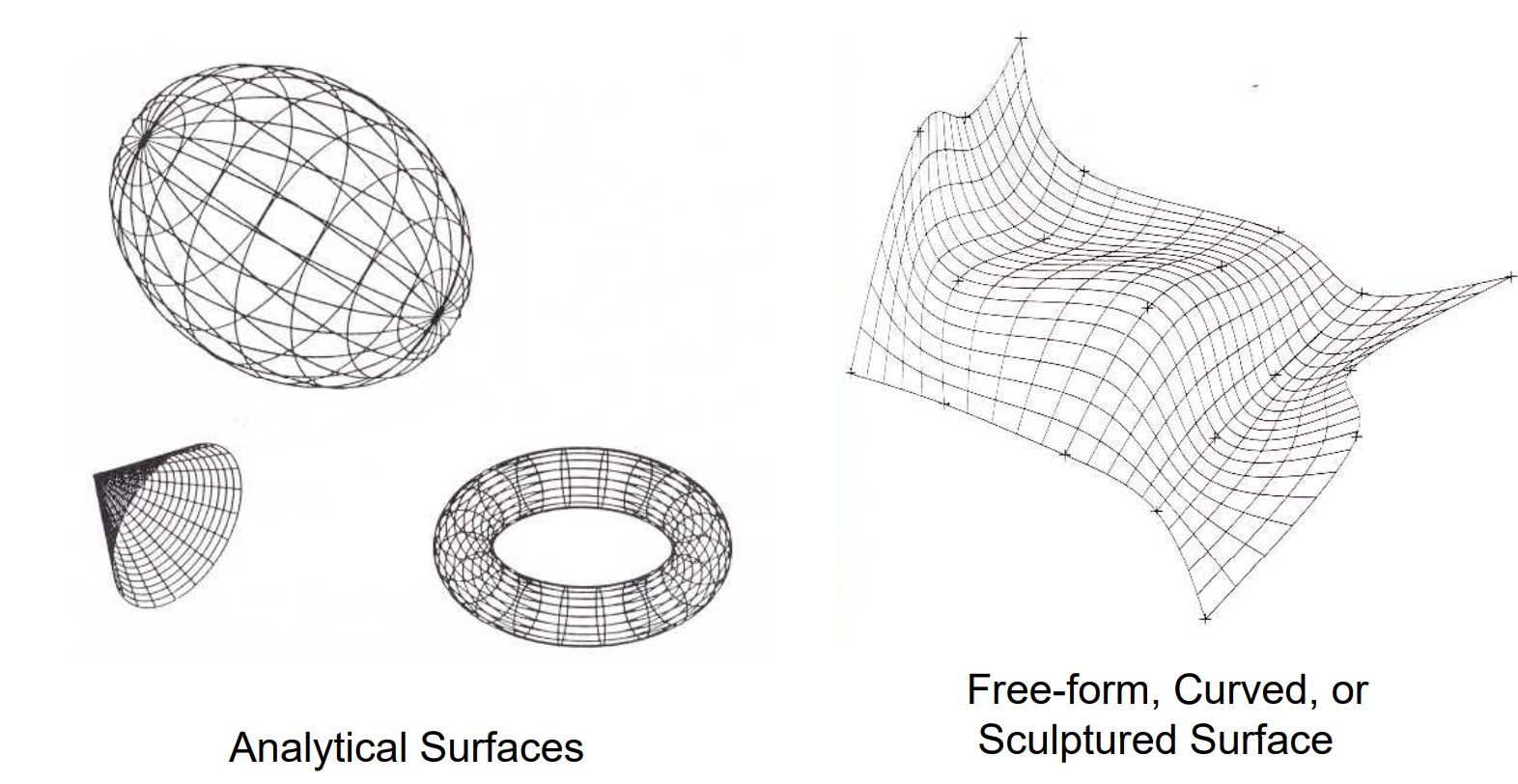# Geometric Modeling Methods

1.0 Introduction

Geometric modeling uses mathematics and knowledge of graphic computers to design objects . There are three main types of geometric models: wire frame models, surface models, and solid models.

2.0 Steps for geometric modeling:

There are three main steps involving the modeling process: 

Step 1: create a basic geometric object using points, lines, curves, etc in a computer-aided design software.

Step 2: transform geometric elements as desired using scaling, rotation, translation.

Step 3: integrate elements of the object to create the final geometric model.

2.1 Wireframe geometric modeling:

Wireframe modeling uses information about vertices and edges to build the object. For example, a rectangle wireframe has 8 vertices and 12 edges. Some advantages of using wireframe are simple construction, less requirement of computer time and memory. However, sers need to input both geometry and topology information.

Besides using points and lines, wireframe modeling utilizes curves. Curves can be constructed using mathematical formulas. Curves can be classified as analytic curves and synthetic curves. With analytical curves, developers can not modify shapes. In contrast, with synthetic curves, developers can interactively modify images.

The Bézier curves are parametric curves commonly used in graphic design.. Curve orders equal the total points minus one (figure 1). Bézier curves are always confined in the convex hull of control points (figure2).

Splines are smooth curves in 2D/3D with specified control points; control points determine the shape of the curves. If a curve passes through all points, it is said to be interpolated. Otherwise, it is said to be approximated. Cubic Bézier curves interpolate the two end points and approximate the two other points. Curve is tangent at P1 and P4.

2.2 Surface modeling

Surface modeling is a set of faces. Surface modeling only stores the geometry. Figure 3 shows some common surfaces.Figure 3: Types of surfaces: Analytical surfaces and sculptured surfaceSource: https://www.engr.uvic.ca/~mech410/old/2_Lecture_Notes/5_Geometric_Modeling.pdf

2.2.1 Bézier surfaces

The Bézier surface of degree (n, m) has a set of (n + 1)(m + 1) control points. Bézier surfaces can be of any degree, but bicubic Bézier surfaces generally provide enough degrees of freedom for most applications. Figure 4 shows the  Bézier surface with its control points. Figure 5 shows some application of the Bezier curves in geometric modeling.

2.3 Solid modeling

Solid modeling stores both geometry and topology information of the object. Some predefined constructive solid geometry are cylinder, cone, sphere, and rectangular prism. Constructive solid geometry follows the boolean operations (figure 6) and the tree model (figure 7). Those are tools to generate a variety of objects from original simple shapes.

2.4 Other techniques:

2.4.1 Sweeping technique can be used to build constructive solid objects with translational and rotational symmetry. The technique is based on two principles: extrusion and revolving.

2.4.2 Boundary representation defines objects based on its spatial boundaries. However. Boundary presentation does not guarantee to form a closed object.

3.0 Conclusion

In this short introduction, beginners of graphic designs can learn about basic techniques in geometric modeling. Learners can do some more research and learn how to implement the Bezier curves and apply it into object designing. For learners who are into mathematics and graphic design, it would be beneficial to invest in a deeper learning about geometric modeling.

Reference

 Design Tech. Computer geometric modeling. [Online]. Available: https://www.designtechsys.com/articles/computer-geometric-modelling. Accessed Nov 13th, 2021.

 Ilya Kantor. (2020). Bezier curve. Javascript.info. [Online]. Available:  https://javascript.info/bezier-curve. Accessed Nov 13th, 2021.

 Wojciech Matusik. Bezier Curves and Splines. MIT OCW. [Online]. Available: https://ocw.mit.edu/courses/electrical-engineering-and-computer-science/6-837-computer-graphics-fall-2012/lecture-notes/MIT6_837F12_Lec01.pdf. Accessed Nov 13th, 2021.

 Wikipedia. Bezier surface. [Online]. Available: https://en.wikipedia.org/wiki/B%C3%A9zier_surface. Accessed Nov 13th, 2021.  Qingnan Zhou. (2018). Mesh Boolean. PyMesh. [Online]. Available: https://pymesh.readthedocs.io/en/latest/mesh_boolean.html. Accessed Nov 13th, 2021.